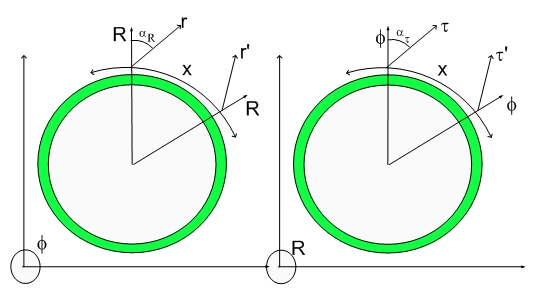# The Passage of TimeThe Passage of Time
Before I forget, I posted a newer version of my theory.

A friend of mine who is very curious about the meaning of time...:) asked me to deliver the details promised in my book...:) Kind of anti-climatic... but I guess I have to oblige...

The problem is simple from the Hypergeometrical point of view... I mentioned that a deformation shown on the two cross-sections of the Hypergeometrical Universe icon correspond to an inertial reference frame with a given velocity v1. That velocity has a corresponding angle alpha1.

Let's just consider what would happen to someone in that reference frame observing something moving at a velocity v2 corresponding to an angle alpha2. This angle is now measured with respect to the inertial reference frame with velocity v1.
As you know angles have to be added according to the equation below
tan(alpha1 + alpha2)=(tan(alpha1)+tan(alpha2))/(1+tan(alpha1)*tan(alpha2))
in the RXYZ cross-section
or the corresponding hyperbolic version
tanh(alpha1 + alpha2)=(tanh(alpha1)+tanh(alpha2))/(1+tanh(alpha1)*tanh(alpha2))
on the PSIXYZ cross-section
This equation means that the addition of angles alpha1 and alpha2 saturates at 45 degrees or the speed of light..

Lorentz transforms are a contrivance due to the way Physics evolved. If the Quantum Lagrangian Principle and the Hyperspherical Shockwave Universe topology were discovered earlier, interactions would naturally not produce any speed higher than the speed of light.

Time affect us through two different processes: a) The first one is through Gravitation/electromagnetism...b) The second is through nuclear decaying processes.

The first kind of processes is very broad... Gravitation and electromagnetism will control the speed of your chemistry, electrochemistry, thoughts...:) the perception of the passage of time etc...:) These processes are controlled by the tiny angles in the 4D space associated with the dilator-dilaton field interaction occurring at each de Broglie step of the Hyperspherical Universe lightspeed expansion. The actual angle change due to interaction also saturates at 45 degrees. This means that the ability of interactions to produce results (change in the state of motion) decreases as v1 tends towards c...

From the point of view of someone sitting in the fabric of space, these angles grow smaller as v1 reaches close to the speed of light. Of course, the tangent formula for angle addition is the basis for the Lorentz transforms. This means that forces become weaker, dynamics slower. Time seems to be passing by quite slowly...:)

In fact, time is passing always at the same speed. The cosmological time doesn't wait for nobody...:) The weakening down of gravitation and electromagnetism results in a slowed down chemistry, electrochemistry, dynamics...Anything traveling close to the speed of light will be frozen...:)which is quite distinct from some funky flow of mysterious time particles...or anything our imaginative mind can conjure up...

That said, one should address the slowing down of the atomic clock...:) How does lifetimes changes with the local deformation of the Fabric of Space...:) Notice that I didn't invoke speed or anything that might bring back the automaton reaction of restricted relativists... :)

Since the particle lifetime also slows down accordingly, the only conclusion one can reach is that nuclear lifetimes are inversely proportional to the deformation associated with the complex dilator (complex deformation coherences). The angle saturation at the speed of light makes any particle to be flattened with the surrounding Fabric of Space.

Serendipitous to some degree...and perhaps just an approximation.

Thus neither Einstein nor anyone else could ask the correct question... which has three items:
a) How many forces are out there??? I say one and if you really pressure me, I might say three (Gravitation,Electrostatics and Magnetism)...which I unified in my paper.
b) How those forces depend upon the local Fabric of Space deformational angle?
c) How particle lifetimes depend upon the local Fabric of Space deformational angle?

Those are trick questions to figure out... The first the one has an unexpected low number of forces...:) The second has, implicit in it, the proposed universe topology and the recast of Newton's equations in terms of FS deformation...

The third requires the creation of an alternative standard model (The Hypergeometrical Standard Model).

No wonder no one knew what time is!!!!

Cheers,

MP

PS- I will soon tell you how one can actuate upon the Fabric of Space ... This is a impressive feat since the Fabric of Space is very thin, embedded in a 4D manifold, traveling at the speed of light...:)
I will give you a hint... if you were living inside a page of a book... How would you find a way to point outwards...:) in the direction of the third dimension...:) Remember that you are a 2D person....:) Quite a feat..:) Please make sure to leave a message if you can figure this out...:)
Then moved the same idea onto the 3D light speed expanding hyperspherical universe... Can you tell where R is poyinting to...:)
Currently unrated

### New Comment

required

required (not published)

optional

required